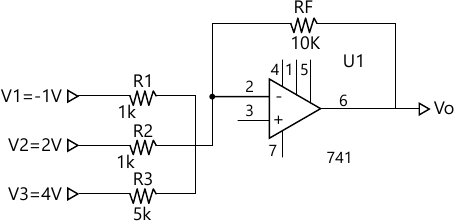MORE IN Basic Electronics Engineering (BXE)
SPPU First Year Engineering (Semester 1)
Basic Electronics Engineering (BXE)
June 2015
Total marks: --
Total time: --
INSTRUCTIONS
(1) Assume appropriate data and state your reasons
(2) Marks are given to the right of every question
(3) Draw neat diagrams wherever necessary

Answer any one question from Q1 and Q2
1 (a) Explain the working of positive clamper with its wavefoRMS.
6 M
1 (b) What is DC load line ? Explain the role of Q-point on DC load line.
6 M

2 (a) Explain the working of positive biased shunt clipper with its input and output wavefoRMS.
6 M
2 (b) Differentiate between CB, CE and CC configurations.
6 M

Answer any one question from Q3 and Q4
3 (a) In shown in the following Fig. let R1 = R2 = 1 kΩ , R3 = 5 kΩ, Rf = 10 kΩ, V1 = -1V, V2 = 2V and V3 = 4V. Calculate Vo.6 M
3 (b) State the IC number for the following two input logic gate:
(i) AND
(ii) NAND
(iii) OR
(iv) NOR
(v) EX-OR
(vi) NOT
6 M

4 (a) Define the following parameters of op-amp:
(i) BW
(ii) PSRR
(iii) CMRR
6 M
4 (b) Draw the explain full adder using two half adder with its truth table.
6 M

Answer any one question from Q5 and Q6
5 (a) With a neat diagram explain the construction and working of LVDT. Give its advantages, disadvantages and applications.
7 M
5 (b) Draw a neat block diagram of a digital thermometer and explain its operation.
6 M

6 (a) Write a short note on Selection criterion for transducers.
7 M
6 (b) Compare:
i) SCR and TRIAC
ii) DIAC and TRIAC
6 M

Answer any one question from Q7 and Q8
7 (a) Draw and explain the electromagnetic spectrum or IEEE frequency spectrum. List its applications.
7 M
7 (b) Compare AM and FM.
6 M

8 (a) What is modulation? Explain AM technique in detail and write Am expression.
7 M
8 (b) Explain the basic structure of mobile phone system.
6 M

More question papers from Basic Electronics Engineering (BXE)